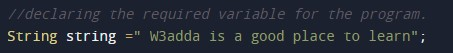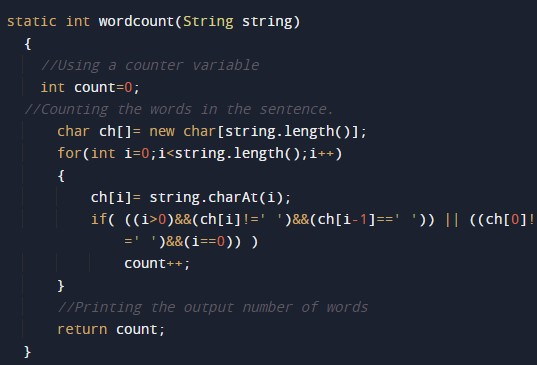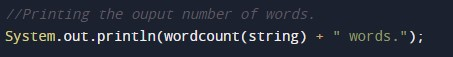# Java program to count number of words in sentence

In this tutorial you will learn about the Java program to count number of words in sentence and its application with practical example.

## Java program to count the number of words in a sentence

In this tutorial, we will learn to create a Java program that will count the number of words in a sentence in Java programming

## Prerequisites

Before starting with this tutorial, we assume that you are the best aware of the following Java programming topics:

• Operators in Java Programming.
• Basic Input and Output function in Java Programming.
• Basic Java programming.
• For loop in Java programming.
• Conditional Statements in Java programming.

## What is a Sentence?

As we all know, a sentence is a collection of characters and words. The word is a collection of alphabets. For string, only one variable is declared which can store multiple values. The String can consist of all the typeable data, it means Digits, Alphabets, Symbols, etc.

## Count the number of words in a sentence

In this program, first, we will declare the input string in the program. Then we will declare the word to be searched in the sentence. Then will find the occurrence of that word in that string. Printing the result if that string is present in the sentence or not.

Let us take the example program from the below code to search for the occurrence of that word.

## Output:-In the above program, we have first initialized the required variable.• txt = It will hold the string value.
• str1 = It will hold the string value.

Count the words in the sentence using a conditional statement.Logic code of the user-defined functionsPrinting output number of words in the sentence.In this tutorial we have learn about the Java program to count number of words in sentence and its application with practical example. I hope you will like this tutorial.